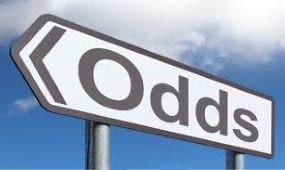# How to Convert Odds

Published: 6th February 2019
Author: Joe Kizlauskas
Last Updated: 6th January 2021# How You Convert Odds

Odds sit at the centre stage of fixed-odds betting which is universally accepted as the most popular way of betting on sports. It is basically a form of wagering against a set of odds on a sporting event set and offered by a bookmaker.

There are three basic types of odds – Fractional Odds, Decimal Odds and Moneyline Odds. Fractional odds are closely connected to the United Kingdom and are historically respected as the initial form first used at race tracks and on the high street. Fractional odds are represented as 5/2, 4/6 or 3 to 2 on etc.

In recent times, however, there is a strong trend in favour of decimal odds simply because they are quite easy to understand, as they are represented in a more understandable and accessible manner – 1.85, 280 etc.

The main difference between the fractional and decimal odds is that the first ones only represent winnings, whereas the latter include the returned stake in addition to the payout. The growing world of online betting has had a hand in the promotion of decimal odds at the expense of fractions, which became quite hard to compute for a regular punter.

Ultimately, the Moneyline Odds are a world of their own and are predominantly used in the United States of America, which is why they are also called American Odds. Moneyline Odds represent the two possibilities with the figure quote presented as either positive or negative. If the figure is positive, the odds are quoting how much money is there to be won on a \$100 wager, whereas the negative prefix marks how much money needs to be wagered in order to win \$100.

## Converting the Odds

Once you have mastered the skill of odds conversion, you will have significantly increased your chances of emerging as winners in the long-run. With the process of converting the odds, it is extremely important to pay attention to implied probability behind the odds, which can be vital when it comes to assessing the potential value of a bet.

Understanding probability is the key and in order to explain it in fewest words possible, it would be enough to say that probability is a measure of chance on a particular event. In other words, it is a number of ways a given event – sports match, roll of a dice, coin toss – can occur divided by the total number of possible outcomes. Further, on below, we demonstrate how to convert odds – fractional and decimal – into implied probability and into one another which is what many bettors find particularly interesting and desired.

## Fractional Odds

As a reflection of the possible return, you will get a particular amount invested on a bet, fractional odds has been the preferred choice since pretty much the invention of online betting. There are many bettors who are not exactly too keen on them as calculating the implied probability and bet value as consequence is unfairly perceived as tedious.

The best way to illustrate it is by an example so let us imagine that a bookie is offering Liverpool at 6/4 betting odds against Manchester City. These odds mean that for every 4 units you bet, there is 6 of them back as profit meaning that if you bet £40 on the Reds, there is a profit of £60 in return, plus your original stake of £40.

If you want to calculate the implied probability of a 6/4 bet on Liverpool, you would do it following the simple formula: Denominator / Denominator + Numerator with the result then multiplied by 100 to express the result as an implied probability percentage.

In this particular case, the Implied probability is calculated as 4 / (6+4), which results in 4 / 10 = 0.4.
Ultimately, multiplied by 100 it gives a percentage of \$40%.

## Decimal Odds

Unlike fractional odds, their European counterparts are reflecting the return on single unit placed. If we are to stick with Liverpool and imagine that Reds are offered at 1.85 to win at Bournemouth, this means that on every £1 bet you would end up winning £0.85 if Reds do win.

Converting the decimal odds into their implied probability is done through a simple calculus which divides 1 by decimal odds. For the 2.00 betting odds, the implied probability is 1 / 2.00 which makes it 0.50 which, multiplied by 100, results in a percentage value of 50%.

Converting Odds from Fractional to Decimal and Vice Versa. Punters find this particular skill rather helpful as it allows them to go through different types of betting odds and comparing them without any issues. The good thing about online betting is that the wonderful world of the Internet has brought forward plenty of online calculators. However, for all the pedestrian bettors who like to get their work done by none other than themselves, here is how to do it.

This is also where implied probability plays a vital role and self-explains it at the crucial part of the puzzle since we would not be able to do the calculus without it. However, once we know the implied probability, the calculation is quite easy. Let us first take the implied value we got from our fractional odds calculus on Liverpool vs Manchester City above – 40%.
The simple math says we need to divide 100 by the Implied probability to get the decimal odds from the fractional ones, which in our particular case stands as 100 / 40 = 2.50

Another example of 50% we got from calculating the implied probability on Liverpool at Bournemouth will have us dividing 100 by Implied probability and deducting 1 from the sum. In other words: (100 / 50) – 1 = 2 – 1 = 1. The given outcome is also known as Evens and is expressed as 1/1 (1 to 1).